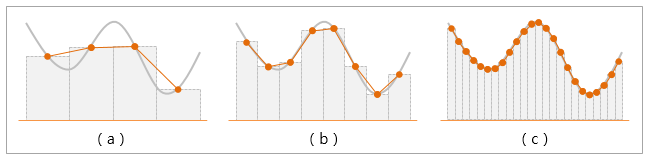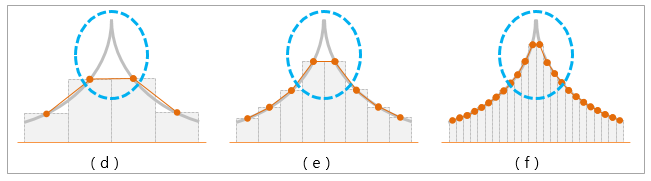Civil Created Edited

# When modeling with beam elements, what is the appropriate mesh size?

## Question

When modeling with beam elements, what is the appropriate mesh size?

As mesh size becomes smaller, it is possible to obtain more accurate results. However, considering the performance of the system, it should be divided into appropriate mesh sizes, and appropriate mesh size is a part where the designers must make their own judgment.

In finite element analysis, the three factors that have the greatest influence on the accuracy of results are mesh size, time step, and element order.

The difference in the accuracy of results depending on the mesh size can be explained by the following figure.In the above figure, (a) represents a case where the mesh size is large, and (c) represents a case where the mesh size is small.

Assuming the gray line is the analytical solution and the orange line is the numerical solution, as seen in the figure, the numerical solution in (a) shows a significant difference from the analytical solution, while (c) produces results that are almost similar to the analytical solution.

Such differences in analysis results due to mesh size can be more pronounced in stress concentration areas.Let's assume that the analytical solution of the resulting moment is the same as the gray line in the figure above.

In areas where concentrated loads are applied, stress is concentrated as shown in the above figure. In such areas, the numerical analysis results of the model with a large mesh size (d) and the model with dense elements (f) show a larger difference compared to other areas.

(Note that after a certain level of element division in Plate/Solid elements, the result converges to a constant value.)

Using finite element analysis software means that you assume that you will represent the actual structure with a finite number of nodes and elements within the limits of the program's performance. The smaller the mesh size, the more accurate the result will be, but it is necessary to divide it into an appropriate mesh size considering the performance of the system, and the appropriate mesh size is a part that designers must judge for themselves.

0